# Python | Arithmetic operations in excel file using openpyxl

• Difficulty Level : Easy
• Last Updated : 19 May, 2021

Prerequisite: Reading & Writing to excel sheet using openpyxl
Openpyxl is a Python library using which one can perform multiple operations on excel files like reading, writing, arithmetic operations and plotting graphs. Let’s see how to perform different arithmetic operations using openpyxl.

Attention geek! Strengthen your foundations with the Python Programming Foundation Course and learn the basics.

To begin with, your interview preparations Enhance your Data Structures concepts with the Python DS Course. And to begin with your Machine Learning Journey, join the Machine Learning - Basic Level Course

• =SUM(cell1:cell2) : Adds all the numbers in a range of cells.

## Python3

 `# import openpyxl module``import` `openpyxl` `# Call a Workbook() function of openpyxl``# to create a new blank Workbook object``wb ``=` `openpyxl.Workbook()` `# Get workbook active sheet ``# from the active attribute.``sheet ``=` `wb.active` `# writing to the cell of an excel sheet``sheet[``'A1'``] ``=` `200``sheet[``'A2'``] ``=` `300``sheet[``'A3'``] ``=` `400``sheet[``'A4'``] ``=` `500``sheet[``'A5'``] ``=` `600` `# The value in cell A7 is set to a formula``# that sums the values in A1, A2, A3, A4, A5 .``sheet[``'A7'``] ``=` `'= SUM(A1:A5)'` `# save the file``wb.save(``"sum.xlsx"``)`
• Output: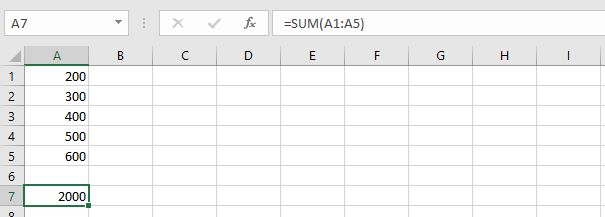• =PRODUCT(cell1:cell2) : Multiplies all the numbers in the range of cells.

## Python3

 `import` `openpyxl` `wb ``=` `openpyxl.Workbook()``sheet ``=` `wb.active` `sheet[``'A1'``] ``=` `2``sheet[``'A2'``] ``=` `3``sheet[``'A3'``] ``=` `4``sheet[``'A4'``] ``=` `5``sheet[``'A5'``] ``=` `6` `# The value in cell A7 is set to a formula``# that multiplies the values in A1, A2, A3, A4, A5 .``sheet[``'A7'``] ``=` `'= PRODUCT(A1:A5)'` `wb.save(``"product.xlsx"``)`
• Output: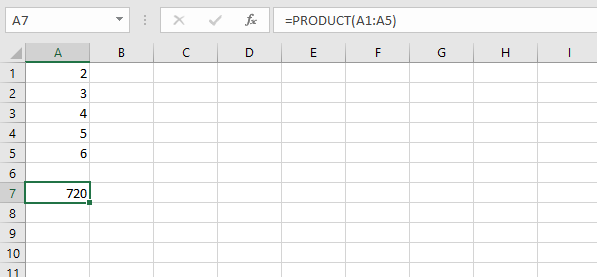• =AVERAGE(cell1:cell2) : It gives the average (arithmetical mean) of all the numbers which is present in the given cell range.

## Python3

 `import` `openpyxl` `wb ``=` `openpyxl.Workbook()``sheet ``=` `wb.active` `sheet[``'A1'``] ``=` `200``sheet[``'A2'``] ``=` `300``sheet[``'A3'``] ``=` `400``sheet[``'A4'``] ``=` `500``sheet[``'A5'``] ``=` `600` `# The value in cell A7 is set to a formula``# that return average of the values in A1, A2, A3, A4, A5 .``sheet[``'A7'``] ``=` `'= AVERAGE(A1:A5)'` `wb.save(``"average.xlsx"``)`
• Output: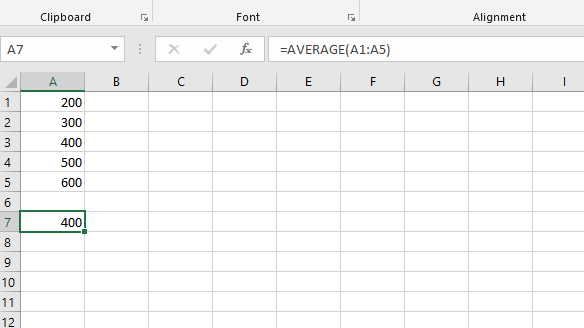• =QUOTIENT(num1, num2) : It returns the integer portion of a division.

## Python3

 `import` `openpyxl` `wb ``=` `openpyxl.Workbook()``sheet ``=` `wb.active` `# The value in cell is set to a formula``# that gives quotient value .``sheet[``'A1'``] ``=` `'= QUOTIENT(64, 8)'``sheet[``'A2'``] ``=` `'= QUOTIENT(25, 4)'` `wb.save(``"quotient.xlsx"``)`
• Output: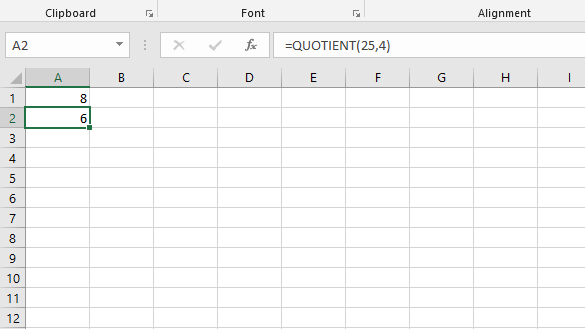• =MOD(num1, num2) : Returns the remainder after a number is divided by the divisor.

## Python3

 `import` `openpyxl` `wb ``=` `openpyxl.Workbook()``sheet ``=` `wb.active` `# The value in cell is set to a formula``# that gives remainder or modulus value.``sheet[``'A1'``] ``=` `'= MOD(64, 8)'``sheet[``'A2'``] ``=` `'= MOD(25, 4)'` `wb.save(``"modulus.xlsx"``)`
• Output: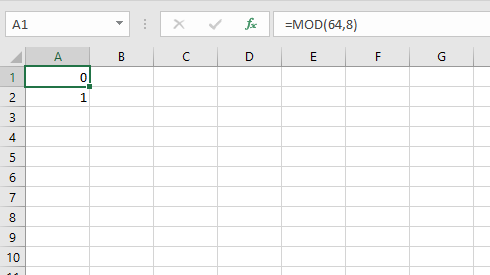• =COUNT(cell1:cell2) : It counts the number of cells in a range that contain the number.

## Python3

 `import` `openpyxl` `wb ``=` `openpyxl.Workbook()``sheet ``=` `wb.active` `sheet[``'A1'``] ``=` `200``sheet[``'A2'``] ``=` `300``sheet[``'A3'``] ``=` `400``sheet[``'A4'``] ``=` `500``sheet[``'A5'``] ``=` `600` `# The value in cell A7 is set to a formula``# that gives counting of number present in the cells.``sheet[``'A7'``] ``=` `'= COUNT(A1:A6)'` `wb.save(``"count.xlsx"``)`
• Output: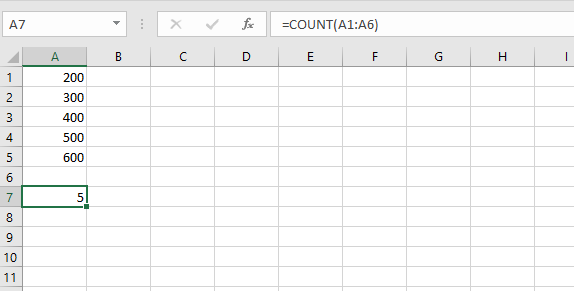My Personal Notes arrow_drop_up